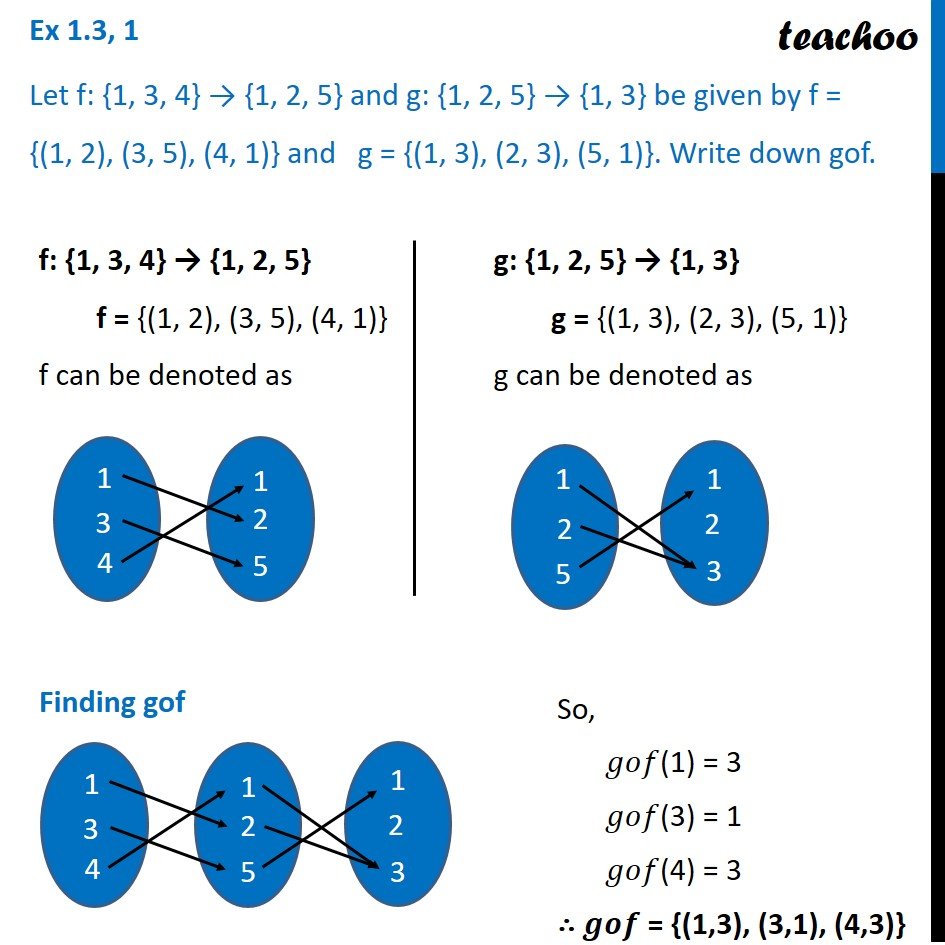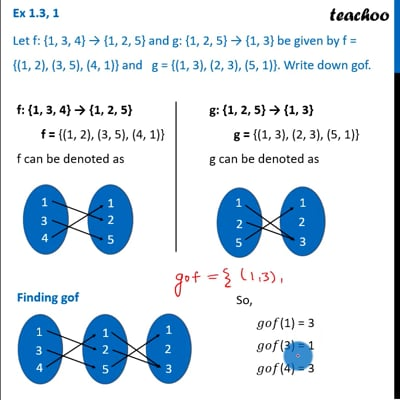Inverse of a function

Chapter 1 Class 12 Relation and Functions
Serial order wiseThis video is only available for Teachoo black usersThis video is only available for Teachoo black users

Learn in your speed, with individual attention - Teachoo Maths 1-on-1 Class

### Transcript

Ex 1.3, 1 Let f: {1, 3, 4} → {1, 2, 5} and g: {1, 2, 5} → {1, 3} be given by f = {(1, 2), (3, 5), (4, 1)} and g = {(1, 3), (2, 3), (5, 1)}. Write down gof. f: {1, 3, 4} → {1, 2, 5} f = {(1, 2), (3, 5), (4, 1)} f can be denoted as g: {1, 2, 5} → {1, 3} g = {(1, 3), (2, 3), (5, 1)} g can be denoted as Finding gof So, 𝑔𝑜𝑓(1) = 3 𝑔𝑜𝑓(3) = 1 𝑔𝑜𝑓(4) = 3 ∴ 𝒈𝒐𝒇 = {(1,3), (3,1), (4,3)}